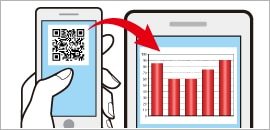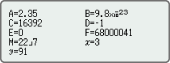# fx-991EX

Close

• The colors may differ slightly from the original.

## fx-991EX

Product Code： C63
GCSE/AS/A Level
Suitable for Cambridge International Examinations GCSE, AS & A Level.
Number of Functions : 552
Natural textbook display
Display expression same as textbook.
Solar & Battery
Solar powered when light is sufficient, battery powered when light is insufficient.

## Features

### Intuitive, easy-to-learn icon displayThe use of icons on the menu screen improves viewability. Selection of the desired function is quick and easy.

### Natural Textbook DisplayInput and display fractions, powers, logarithms, roots, and other mathematical formulas and symbols just as they appear in textbooks.

### List display function for thorough, speedy learningVariables and statistical calculation results stored in memory can be displayed in lists. There’s no need to recall and confirm individual values as with previous models.

### Easy-to-understand English messages and interactive formatNearly all English words are displayed on the screen in unabbreviated form. Interactive menu display realizes more intuitive operation.

### High computational competence for performing even advanced mathematicsClassWiz contains calculation functions that support even advanced mathematical operations, including spreadsheet calculations, 4 × 4 matrix calculations, calculation of simultaneous equations with four unknowns and quartic equations, and advanced statistical distribution calculations.

### Online Visualization Service Using QR CodeGenerate QR codes of equations input into the calculator by a simple operation. Graphs and other graphics can be displayed on smartphone or tablet screens.

### A comprehensive set of advanced functions unique to CASIO scientific calculatorsUseful for learning statistics. Up to 5 columns × 45 rows (maximum of 170 data items)

#### Matrix calculationsPerform calculations with matrices of up to 4 rows and 4 columns.

#### Vector calculationsPerform calculations using up to four third-order vectors stored in memory.

#### Multiline displayFormulas are automatically wrapped to multiple lines. *
*In linear input mode

#### Integration calculationsPerform integration calculations in advanced mathematics.

#### Differential calculations>
Perform differential calculations in advanced mathematics.

#### Equation calculationsCalculation of simultaneous equations in 2 to 4 unknowns and high-degree equations of second to fourth degree

#### Inequality calculationsSolve second-degree to fourth-degree inequalities.Perform calculations involving normal distributions, binomial distributions, and Poisson distributions.

#### Ratio calculationsPerform ratio calculations such as A:B=X:D.

#### Metric conversionsSelect units from the Metric Conversion Command List.

#### Scientific constantsSelect scientific constants from the Scientific Constant Table.

#### Variables listOne-touch list display

#### Statistics listDisplays six rows at once.

#### Digit separatorSeparation every three digits makes even large numbers easy to read.

#### Engineering symbolsPerform engineering calculations that include symbols such as k, M, m, and n.

## Specifications

• Non Programmable

•GCSE/AS/A Level Suitable for Cambridge International Examinations GCSE, AS & A Level.

•Number of Functions : 552

•Natural textbook display Display expression same as textbook.

•10 + 2 digits 10-digit mantissa + 2-digit exponential display.

•Dot matrix display High-resolution screen provides beautiful looking graphs every time.

•Icon menu Specify the operation you want to perform by selecting an icon or inputting a number.

•Multi-replay Quick and easy recall of previously executed formulas for editing and re-execution.

•Solar & Battery Solar powered when light is sufficient, battery powered when light is insufficient.

•Plastic keys Designed and engineered for easy operation.

• Basic Statistics Standard statistics functions such as Mean, SUM, Standard Deviation, and Regression

• Ratio

• Basic Mathematical Functions Trigonometric, Exponential logarithmic, etc.

• Table Generation You can create a numerical table based on that expression by registering a function expression. In addition, graph functions can be represented by graphs.

• Intermediate Statistics More statistical functions such as Paired-variable statistical calculation, Quartiles and List display functions for speedy and thorough learning .

• Scientific Constants You can use constant symbols used in physics, science classes.

• Vector

• Advanced Statistics Support high level statistics by 7 kinds of advanced distribution calculation.

• Inequality

•List based STAT-data editor Viewing and editing of input data in list format, showing data groups (x-data, y-data, frequency) and surrounding data.

• Differentiation

• Non Graphing

• Matrix

• Equation

• Integration

• High Resolution We are choosing a model with higher definition in our non-programmable model and a higher definition model with Programmable model.

• Complex number calculation

•Metric conversion function You can convert a number in one unit to a number in a different unit.

• QR Code You can see graphs on smartphones etc. using QR Code.